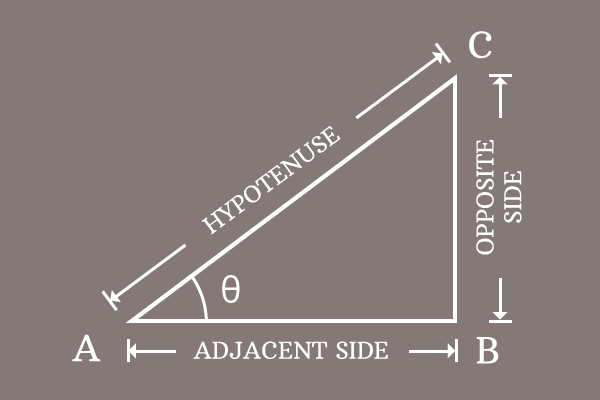# Sine

A term that represents a ratio of lengths of opposite side to hypotenuse at an angle of a right triangle is called the sine.

## Introduction

Sine is a name and it is used to represent the ratio of lengths of opposite side to hypotenuse at a particular angle in a right triangle. It is written in ratio form and also written as sine with angle alternatively.

### Ratio

The value of sine at an angle is calculated by the ratio of lengths of opposite side to hypotenuse.

$\dfrac{Length \, of \, Opposite \, side}{Length \, of \, Hypotenuse}$

So, sine is called as a trigonometric ratio generally.

### Function

Alternatively, the value of sine at an angle is expressed mathematically by writing sine in its short form $\sin$ and then corresponding angle of the right triangle.

For example, if angle of right triangle is denoted by $x$, then sine of angle $x$ is written as $\sin{x}$ in trigonometry. $\sin{x}$ is a function form. So, it is usually called as sin function in mathematics.

Thus, sin functions like $\sin{A}$, $\sin{\alpha}$, $\sin{\beta}$, and etc. are defined in mathematics.

### Mathematical form

$\Delta BAC$ is a right angled triangle and its angle is theta ($\theta$).sine of angle is written as $\sin{\theta}$ in this case.

$\sin{\theta} \,=\, \dfrac{Length \, of \, Opposite \, side}{Length \, of \, Hypotenuse}$

It is used as a formula to calculate the value of sine at any angle of the right triangle.

In this example, $BC$ is length of opposite side (perpendicular) and $AC$ is length of hypotenuse.

$\,\,\, \therefore \,\,\,\,\,\, \sin{\theta} \,=\, \dfrac{BC}{AC}$

#### Values

The list of exact values of sine functions in fraction and decimal forms in a table with proofs.

Latest Math Topics
Jun 26, 2023
Jun 23, 2023

Latest Math Problems
Jul 01, 2023
Jun 25, 2023
###### Math Questions

The math problems with solutions to learn how to solve a problem.

Learn solutions

Practice now

###### Math Videos

The math videos tutorials with visual graphics to learn every concept.

Watch now

###### Subscribe us

Get the latest math updates from the Math Doubts by subscribing us.